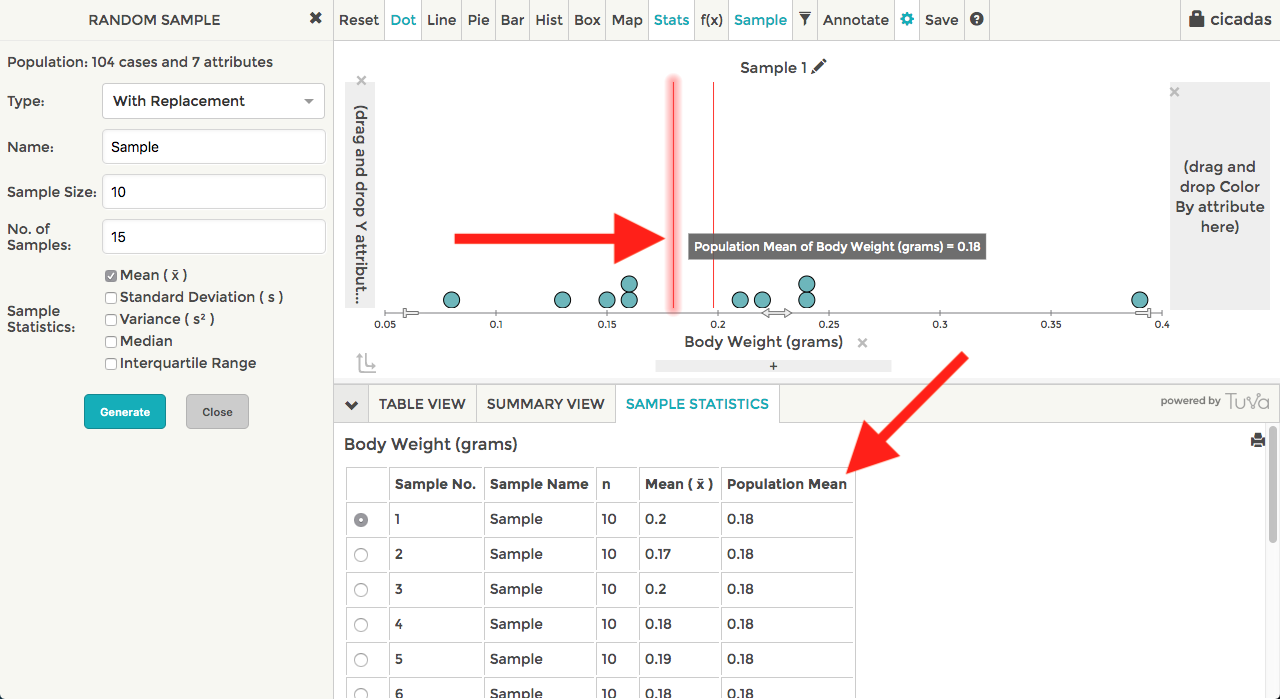# How to Sample a Population with Tuva

Tuva treats each dataset as a population when generating samples. When you're browsing the list of datasets in the content library, each of these datasets can serve as a population.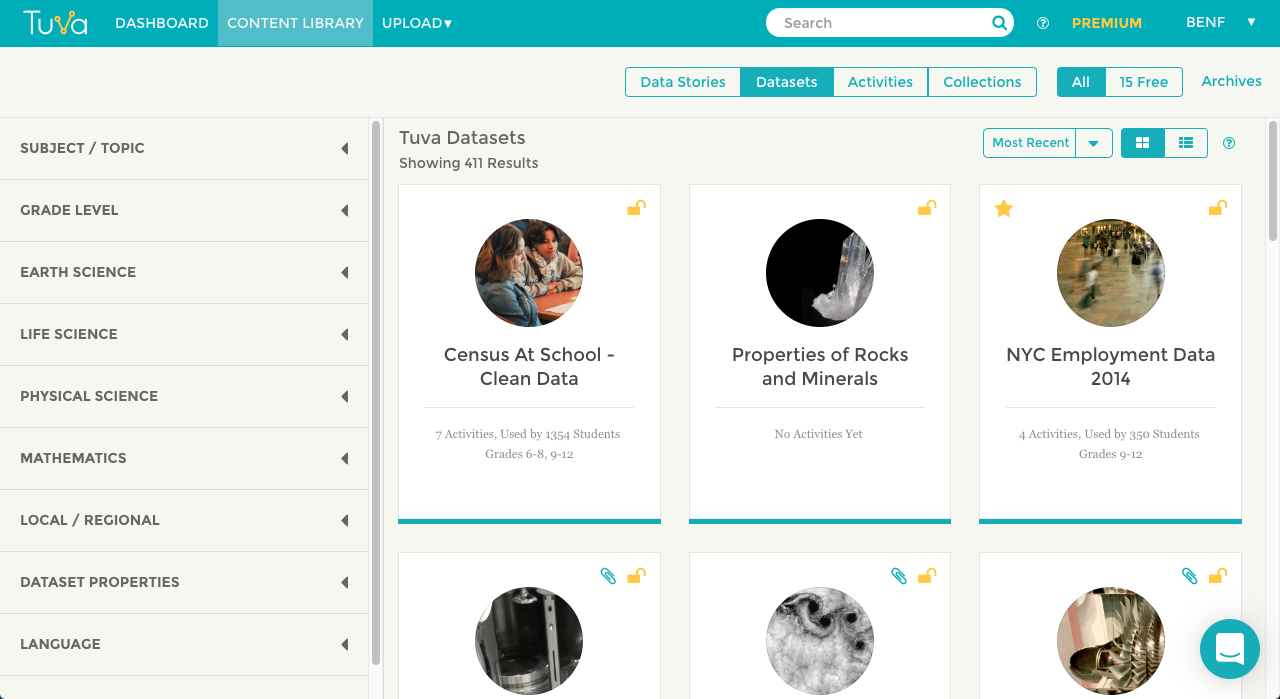Once you select a dataset, you can follow the steps below to generate samples.

## Step 1: Add a Numeric Attribute to either the X- or Y-axis

In the following screenshots, we're working with the Cicadas dataset. When sampling a dataset on Tuva, you need to sample with respect to a numerical attribute. In the screenshot below, you can see we've placed Body Weight (grams) on the X-axis.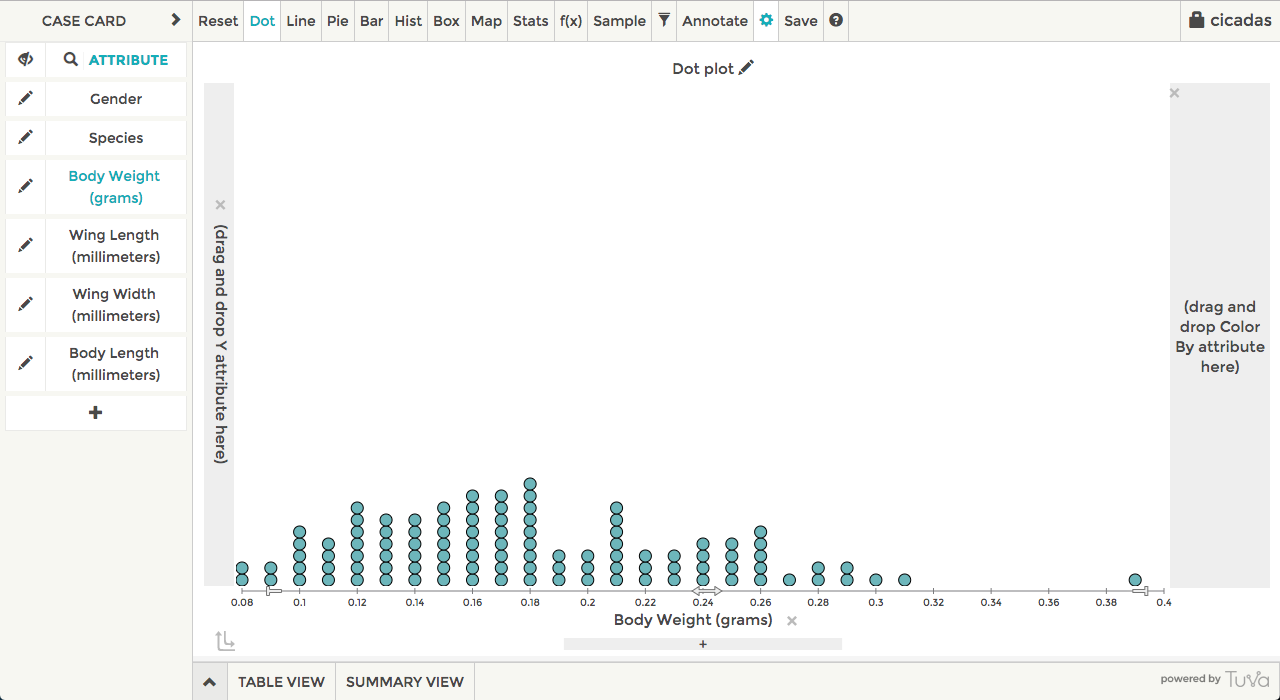## Step 2: The Sampling Panel

To open the sampling panel, we'll need to click the Sample button in the toolbar.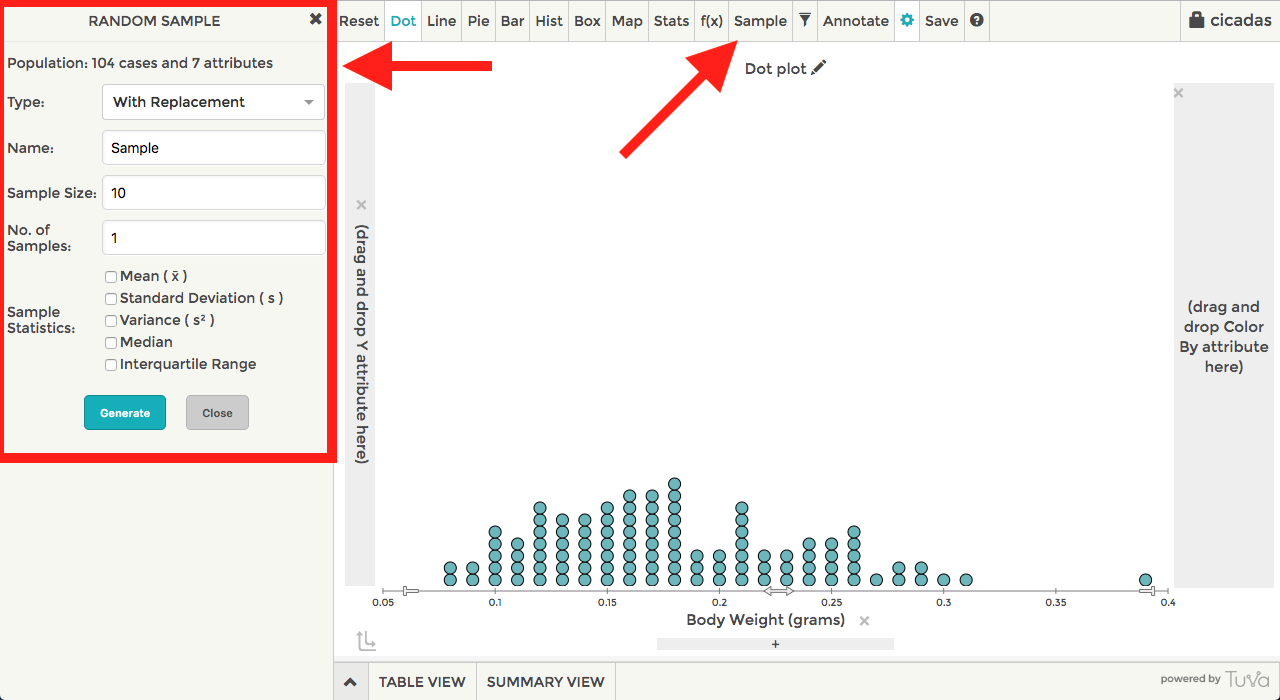You'll then be presented with a series of options: type, name, sample size, number of samples, and sample statistics.

• Type: There are two types of samples you may generate, either with replacement or without replacement.
• Name: This is the name of the sample you want to generate. The name will appear in the plotting area and in the sample statistics table after you generate a sample.
• Sample Size: The sample size is the number of cases you're going to pick from your population.
• Number of Samples: You may generate multiple samples in a single go. Changing the number of samples to a value greater than 1 will allow you to explore variability in the sample statistics.
• Sample Statistics: The sample statistics should be picked before generating a sample. After clicking generate, you will see the selected statistics appear in the plotting area and also in the Sample Statistics table.

## Step 3: Sample Statistics Table

After clicking Generate, a few things will happen. First, the Sample Statistics will appear below the plotting area, which will show you the current sample you're looking at and also list the values for any statistics you've selected. Then, the plot will update to visually show you the cases in your sample and also show any statistics for the specific sample.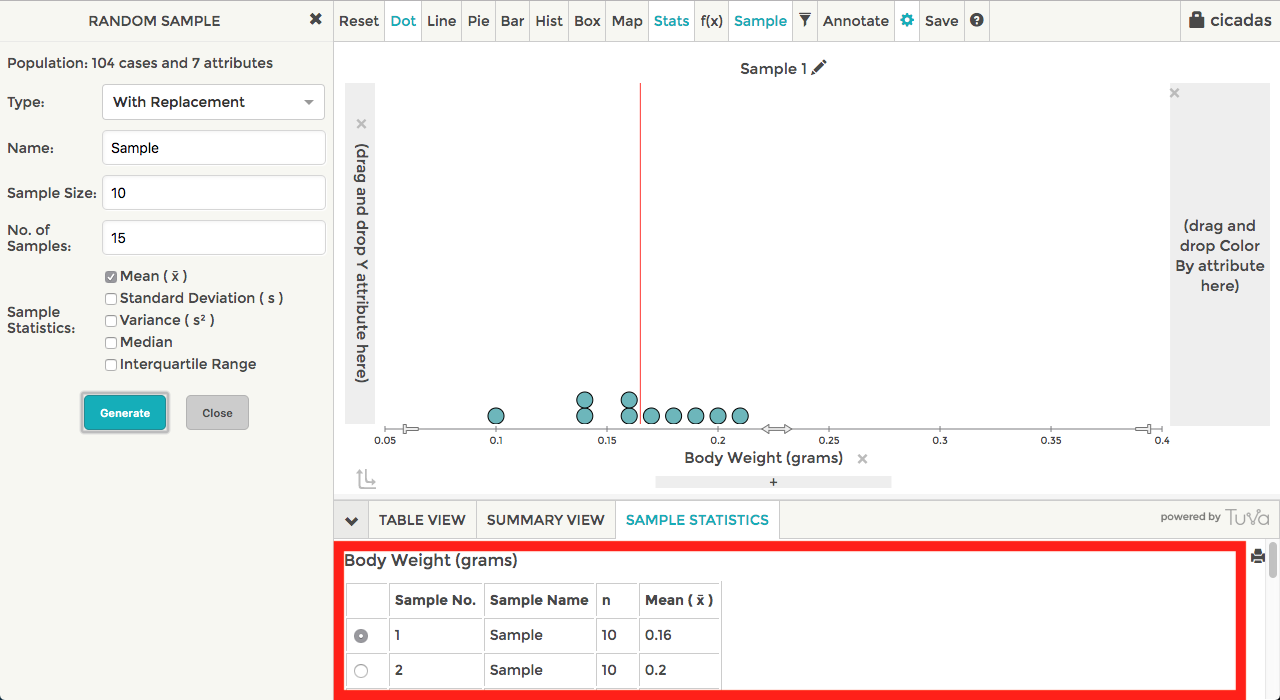You may also expand the Sample Statistics table by clicking and dragging the line separating the plot and the table, so you may view more of the statistics.Also notice the radio buttons in the left hand column of the Sample Statistics table. Clicking on a radio button will update the plot to show the selected sample.

If you're interested in plotting the Sample Statistics table as a way to explore the variability between the sample statistics in comparison to the population, you may copy and paste the table into a spreadsheet. Then, reimport the spreadsheet into Tuva to allow you to visualize the variability with the Tuva Tools.

## Step 4: Population Parameters

Selecting the population parameter will allow you to compare a set of the generated samples to the population. Population parameters are available via the Stats menu.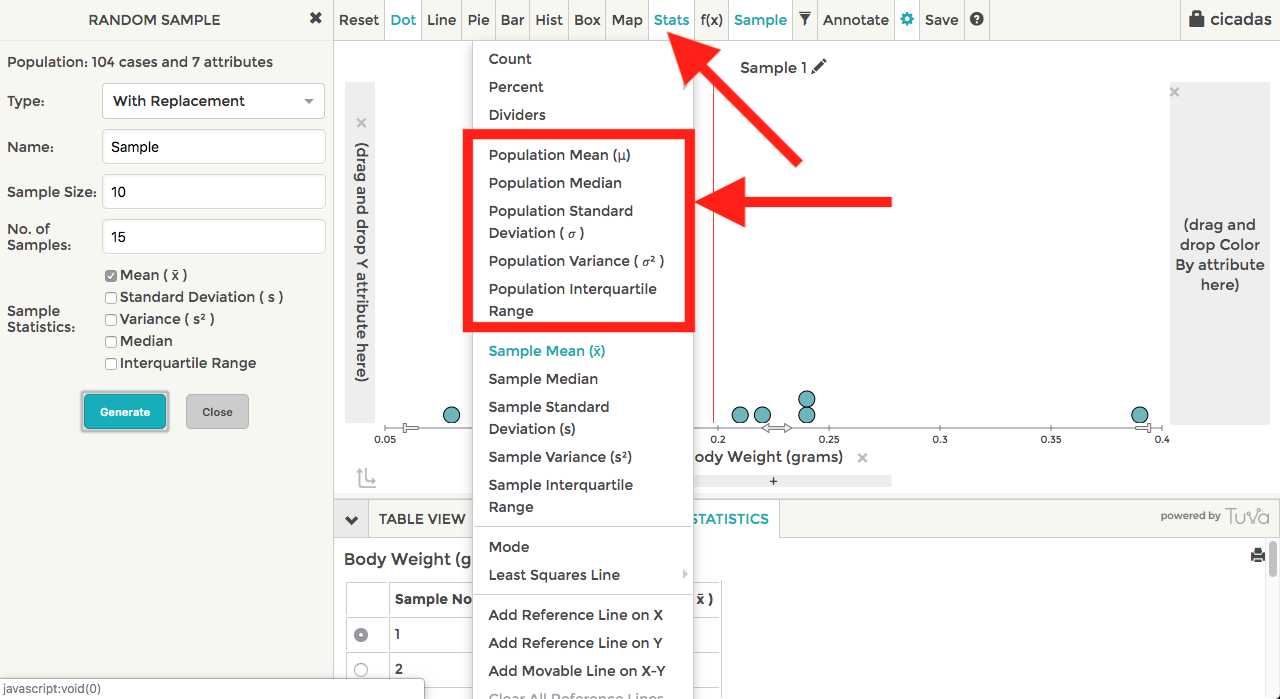After selecting the population parameter, it will appear both in the plot and also the sample statistics table below the plot.